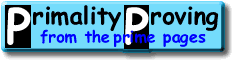Finding primes & proving primality 3.3: Combined TestsHome > Primality Proving > Chapter Three > Combined Tests

In previous sections we have pointed out if the factored portion of n-1 or of n+1 is larger than the cube root of n, then we can prove n is prime.  In this section we discuss the case that the product of these two factored portions is greater than the cube root of n, then we can prove n prime using a combined test.  (If we can not find enough factors to prove n prime this way, then we must use the generalized tests of the following chapter.)

Let n > 1 be an odd integer.  Let n-1 = F1R1 and n+1 = F2R2 where F1 and F2 are completely factored, and gcd(F1,R1) = gcd(F2,R2) = 1.  The two types of tests we have applied to n in the previous sections are as follows.

Condition I.  For each prime p dividing F1 there is an integer a such that
• an-1 ≡ 1 (mod n), but
• gcd(a(n-1)/p-1, n) = 1.
Condition II.  Let (d|n) = -1.  For each prime p dividing F2 there is a Lucas sequence with discriminant d such that
• U(n+1) ≡ 0 (mod n), and
• gcd(U((n+1)/p), n) = 1.

Pocklington's theorem tells us that if (I) is true, then each prime factor q of n has the form k·F1+1.  About 60 years later Morrison proved that if (II) held, then each prime factor q of n has the form k·F2±1 [Morrison75].  Together these give us the following:

Combined Theorem 1:  Suppose n, F1, F2, R1, R2 are as above and conditions (I) and (II) are satisfied.  If n < max(F12F2/2 , F1F22/2), then n is prime.

Proof.  Let q be a prime factor of n and let n = mq.  From Condition I we have that q ≡ 1 (mod F1), so since n is 1 (mod F1), so is m.  From Condition (II) we know q = ±1 (mod F2), so since n is -1 (mod F2), either q or m is 1 (mod F2).  We may assume that m = 1 (mod F2), because if every prime factor q of n was 1 (mod F2), we'd have the contradiction that n ≡ 1 (mod F2).  Finally gcd(F1,F2)=2, so we can combine these to get that m ≡ 1 (mod F1F2/2). So for n to be composite we must have both

• n qm >  ( 1 + F1)(1 + F1F2/2)  >  F12F2/2, and
• n  =  qm  >  (-1 + F2)(1 + F1F2/2)  >  F1F22/2.

This completes the proof of the theorem.

### Adding in a factoring bound

Sometimes, if n is small enough that we have almost enough factors to use the above (or similar) results, it can be helpful to bring in information about how far we have tried to factor n±1.  Suppose, for example, that all of the prime factors of R1 and R2 are greater than B.  Next apply the conditions above to R1 and R2

Condition III.  There is an integer a such that
• an-1 ≡ 1 (mod n), but
• gcd(a(n-1)/R1 -1, n) = 1.
Condition IV.  Let (d|n) = -1.  There is a Lucas sequence with discriminant d (same d as used in condition II) such that
• U(n+1) ≡ 0 (mod n), and
• gcd(U((n+1)/R2), n) = 1.

These two conditions inform us respectively that every prime factor q of n has the form k·u+1 where u is a prime factor of R1; and every prime factor q also has the form k·v±1 where v is a prime factor of R2.  (Note that the factors u and v are dependent on q.)  Of course u and v must each be larger than the factoring bound B.  With the above notation we can now state our final classical theorems.  (For the first the proof is virtually identical to the proof above.)

Combined Theorem 2:  Suppose n, F1, F2, R1, R2, B are as above and conditions (I) through (IV) are satisfied.  Define integers r and s by R1 = sF2/2 + r with 0 < r < F2/2.  If
n < max(B·F1 + 1, B·F2 - 1) (B2F1F2/2 + 1)
then n is prime.
Combined Theorem 3:  Suppose n, F1, F2, R1, R2, B are as above and conditions (I) through (IV) are satisfied.  Again define integers r and s by R1 = sF2/2 + r with 0 < r < F2/2.  If for some integer m
n < (m·F1F2 + r·F1 + 1) (B2F1F2/2 + 1)

then either n is prime or kF1F2 +  rF+ 1 divides n for some non-negative integer k < m.

Both of these results (and more) can be found in "the" paper on the classical results: [BLS75].  Another excellent source on these theorems and their extensions is the excellent text H. Williams "Édouard Lucas and Primality testing" [Williams98]

How much further can we go?  It is possible to consider higher powers such as the factors of

n6 -1 = (n - 1)(n2 + n + 1)(n + 1)(n2 - n + 1).

(See [Williams78] for the theory and examples of these techniques).  But the cost in terms of mathematical complication is very high.  So in practice adding a few terms such as n2+n+1 or n2-n+1 is rarely worth the effort.  Rather it makes sense to just move on to the general primality proving methods of the next chapter.

 [ next page | previous page ] Copyright © 2023 PrimePages (Originally written by Chris Caldwell)